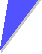# Exercise 5.1.3 PolarizationA light beam with intensity I0 passes through one ideal polarizer. How does the intensity relate to the electrical field strength? The incoming ("input") light beam is unpolarized. How large is the intensity at the output? Does this intensity change if you rotate the polarizer around the axis coinciding with the propagation direction of the light = optical axis? The incoming light beam is 100 % linearly polarized. How large is the intensity on the output as a function of the angle between polarization direction of the light and polarizing direction of the polarizer.A light beam with intensity I0 first passes through one ideal polarizer, and then through a second one. Both polarizers can be rotated freely around the optical axis. The light beam is unpolarized. How large is the intensity on the output if both ideal polarizers are in parallel? The light beam is unpolarized. How large is the intensity on the output if the ideal polarizers are "crossed", i.e. their polarization directions are at right angles? The light beam is 100 % linearly polarized. How large is the intensity of the output as a function of the variable angle a between the two polarizing directions of the polarizers and the fixed angle b between the polarization direction of the light and the first polarizer it encounters? Note that in this case you rotate the second polarizer. Does the result for the question above change if you rotate the first polarizer and keep the second one at the fixed angle b? Is for all of the above the direction of the light paths always reversible as stated before?Now consider a system with two fixed crossed polarizers and a third one that can be rotated in between the two crossed ones. The incoming light beam is unpolarized. How large is the intensity of the output as a function of the variable angle a between the first (fixed) and the third polarizer that can be rotated? The incoming light beam is 100 % linearly polarized. How large is the intensity on the output as a function of the variable angle a between the first (fixed) and the third polarizer (can be rotated) considering that the angle b between the incoming light polarization and the polarization direction of the first polarizer is fixed at a value b?Good schematic drawings with proper values at the axes are sufficientLink to the solution5.1.4 Energy Flow, Poynting Vector and PolarizationSolution to Exercise 5.1-3: Polarization